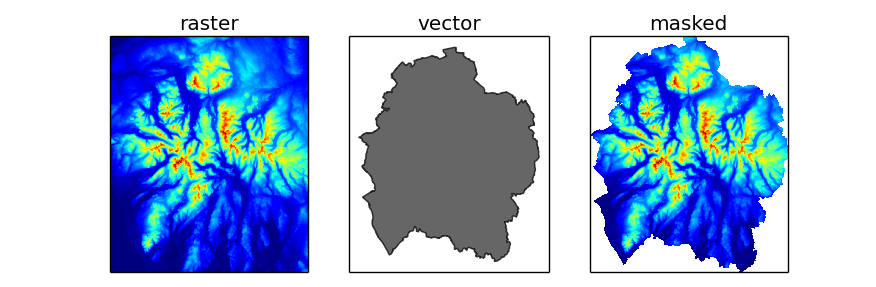Masking Rasterio Layers with Vector Features

Posted on

This post shows how to extract data from a raster only where it intersects a vector feature using Rasterio. Fiona is used to read the vector data, and Shapely is used only as a convenience to calculate the feature’s bounding box.

GDAL provides a function to “burn” vector shapes into rasters (i.e., to rasterize the geometries). This functionality is accessed from Rasterio using the rasterize function. This tool can be used to create a raster mask for another raster layer.

The example below uses two layers: national_parks.shp, a vector layer containing polygon features representing national park boundaries; and elevation.tif, a raster layer representing the elevation above sea level in metres. For each feature in the vector layer, the raster data is extracted using a window, then vector geometry is rasterized and used to mask the raster data. The result is a numpy masked array. SciPy provides the scipy.stats.mstats module which contains various statistical functions that work with masked arrays, but for this example only the min and max methods of the array itself have been demonstrated.Contains Ordnance Survey data © Crown copyright and database right 2014

from __future__ import print_function

import numpy
import numpy.stats.mstats
import fiona
import rasterio
import rasterio.features
from affine import Affine
from shapely.geometry import shape

with fiona.open('national_parks.shp', 'r') as vector, \
rasterio.open('elevation.tif', 'r') as raster:

for feature in vector:
# create a shapely geometry
# this is done for the convenience for the .bounds property only
geometry = shape(feature['geometry'])

# get pixel coordinates of the geometry's bounding box
ul = raster.index(*geometry.bounds[0:2])
lr = raster.index(*geometry.bounds[2:4])

# read the subset of the data into a numpy array
window = ((lr, ul+1), (ul, lr+1))
data = raster.read_band(1, window=window)

# create an affine transform for the subset data
t = raster.affine
shifted_affine = Affine(t.a, t.b, t.c+ul*t.a, t.d, t.e, t.f+lr*t.e)

# rasterize the geometry
[(geometry, 0)],
out_shape=data.shape,
transform=shifted_affine,
fill=1,
all_touched=True,
dtype=numpy.uint8)

# create a masked numpy array

# display some statistics
name = feature['properties']['NAME'].title()
print('{}\nRange: {:.1f} to {:.1f}'.format(
name,